# HTML5 Canvas Line

## Draw Lines

To draw a line using HTML5 Canvas is simple, just like draw a line on a paper, define a path, and then fill the path. See the following steps :

• Resets the current path using beginPath() method.
• Let move the drawing cursor to start point to create a new subpath using moveTo(x,y) method.
• Now use lineTo(x, y) method, which adds a new point and connects this point to the starting point by using a straight line.
• Both the above methods accept x and y parameters which tell it exactly where you want to draw the line.
• Finally use stroke() method to make the line visible.

Pictorial Presentation: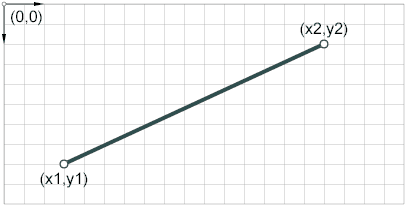Example : Draw a simple Line

The following code will draw a simple line from (10,45) to (180,40).

Output :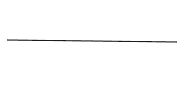``````<!DOCTYPE html>
<html>
<meta charset=utf-8 />
<title>Draw a line</title>
<body>
<canvas id="DemoCanvas" width="500" height="200"></canvas>
<script>
var canvas = document.getElementById('DemoCanvas');
//Always check for properties and methods, to make sure your code doesn't break in other browsers.
if (canvas.getContext)
{
var context = canvas.getContext('2d');
// Reset the current path
context.beginPath();
// Staring point (10,45)
context.moveTo(10,45);
// End point (180,47)
context.lineTo(180,47);
// Make the line visible
context.stroke();
}
</script>
</body>
</html>``````

Example : Draw horizontal and vertical lines

The following code example uses the moveTo and lineTo methods to incrementally draw horizontal and vertical lines across the canvas.

Output: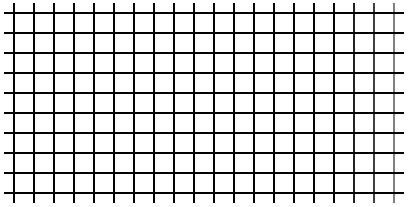``````<!DOCTYPE html>
<html>
<meta charset=utf-8 />
<title>Draw a line</title>
<body>
<canvas id="DemoCanvas" width="400" height="400"></canvas>
<script>
var canvas = document.getElementById('DemoCanvas');
if (canvas.getContext)
{
var ctx = canvas.getContext("2d");
for (i = 10; i < 200; i += 20)
{
ctx.moveTo(0, i);
ctx.lineTo(canvas.width, i);
ctx.stroke();
}
for (i = 10; i <400; i += 20)
{
ctx.moveTo(i, 0);
ctx.lineTo(i,canvas.width/2);
ctx.stroke();
}
}
</script>
</body>
</html>``````

## Line width

The lineWidth property gives the width (in pixels) of lines. The property value is a positive number (default value 1). On setting, zero, negative, and NaN values must be ignored, leaving the value unchanged. The following example draws a series of lines by using increasing values (1 to 12) for the lineWidth property.

Output: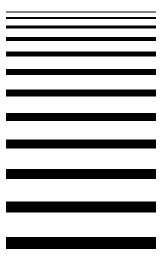``````<!DOCTYPE html>
<html>
<meta charset=utf-8 />
<title>Draw lines of various width</title>
<body>
<canvas id="DemoCanvas" width="400" height="400"></canvas>
<script>
var canvas = document.getElementById('DemoCanvas');
if (canvas.getContext)
{
var context = canvas.getContext("2d");
context.beginPath();
context.moveTo(50, 10);
context.lineTo(50, 200);
context.lineWidth = 5;
// set line color
context.strokeStyle = 'red';
context.stroke();
context.beginPath();
context.moveTo(100, 10);
context.lineTo(100, 200);
context.lineWidth = 5;
// set line color
context.strokeStyle = 'green';
context.stroke();
context.beginPath();
context.moveTo(150, 10);
context.lineTo(150, 200);
context.lineWidth = 5;
// set line color
context.strokeStyle = '#2E1919';
context.stroke();
context.stroke();
context.beginPath();
context.moveTo(200, 10);
context.lineTo(200, 200);
context.lineWidth = 5;
// set line color
context.strokeStyle = '#3B4839';
context.stroke();
}
</script>
</body>
</html>
``````

## Color Lines

To draw color lines you can use strokeStyle property, the default color is black. Syntax of the property is object.strokeStyle = color. Here is an example :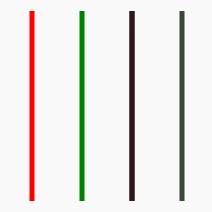``````<!DOCTYPE html>
<html>
<meta charset=utf-8 />
<title>Color Lines</title>
<body>
<canvas id="DemoCanvas" width="400" height="400"></canvas>
<script>
var canvas = document.getElementById('DemoCanvas');
if (canvas.getContext)
{
var context = canvas.getContext("2d");
context.beginPath();
context.moveTo(100, 10);
context.lineTo(100, 200);
context.lineWidth = 5;
// set line color
context.strokeStyle = '#808000';
context.stroke();
}
</script>
</body>
</html>
``````

## Line Cap

lineCap property is used to gets or sets the current line cap style. There are three cap styles :

• butt : Default. A flat edge is put perpendicular to each end of the line with no cap added.
• round : A semicircle or rounded end cap is added to each end of the line.
• square : A square end cap is added to each end of the line.

Here is an example :

Output :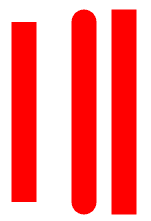``````<!DOCTYPE html>
<html>
<meta charset=utf-8 />
<title>Line Cap</title>
<body>
<canvas id="DemoCanvas" width="400" height="400"></canvas>
<script>
var canvas = document.getElementById('DemoCanvas');
if (canvas.getContext)
{
var context = canvas.getContext("2d");
// butt line cap (first line)
context.beginPath();
context.moveTo(20,20);
context.lineTo(20,200);
context.lineWidth = 25;
context.strokeStyle = '#ff0000';
context.lineCap = 'butt';
context.stroke();

// round line cap (second line)
context.beginPath();
context.moveTo(80,20);
context.lineTo(80,200);
context.lineWidth = 25;
context.strokeStyle = '#ff0000';
context.lineCap = 'round';
context.stroke();

// square line cap (third line)
context.beginPath();
context.moveTo(120,20);
context.lineTo(120,200);
context.lineWidth = 25;
context.strokeStyle = '#ff0000';
context.lineCap = 'square';
context.stroke();
}
</script>
</body>
</html>``````

## Line Join

lineJoin property is used to get or set the type of corner that is created when two lines join. There are three values :

• bevel : A filled triangle connects the two lines that are joined, creating a beveled corner.
• round : Lines join with a rounded corner.
• square : Default. Lines join with a smoothly mitered corner.

Here is an example :

Output :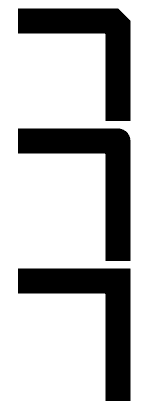``````<!DOCTYPE html>
<html>
<meta charset=utf-8 />
<title>Line Joining</title>
<body>
<canvas id="DemoCanvas" width="400" height="400"></canvas>
<script>
var canvas = document.getElementById('DemoCanvas');
if (canvas.getContext)
{
var ctx = canvas.getContext("2d");
var lStart = 50;
var lEnd = 200;
var yStart = 20;
ctx.beginPath();
ctx.lineWidth = 25;
// Use a bevel corner.
ctx.lineJoin = "bevel";
ctx.moveTo(50, 20);
ctx.lineTo(150, 20);
ctx.lineTo(150, 120);
ctx.stroke();
// Use a round corner.
ctx.beginPath();
ctx.lineJoin = "round";
ctx.moveTo(50, 140);
ctx.lineTo(150, 140);
ctx.lineTo(150, 260);
ctx.stroke();
// Use a miter.
ctx.beginPath();
ctx.lineJoin = "miter";
ctx.moveTo(50, 280);
ctx.lineTo(150, 280);
ctx.lineTo(150, 400);
ctx.stroke();
}
</script>
</body>
</html>``````

See the Pen html css common editor by w3resource (@w3resource) on CodePen.

Previous: HTML5 Canvas Tutorial
Next: HTML5 Canvas arcs tutorial

﻿